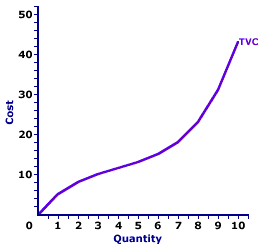Thursday  August 18, 2022
 AmosWEB means Economics with a Touch of Whimsy!YIELD CURVE: A curve plotting the yields (or returns) on securities with different maturity lengths. The standard yield is for U.S. Treasury securities with lengths ranging from 90 days to 30 years. The five maturity lengths are usually 90 day, 180 day, 2 year, 5 year, 10 year, and 30 year. The shape and slope fo the yield curve indicates the state of the economy and what's likely to come. A normal yield curve has a slight positive slope, with slightly higher yields for longer maturity securities. A steep yield curve suggests the end of a contraction and beginning of an expansion. An inverted, or negatively sloped yield curve is the sign of an upcoming contraction.TOTAL VARIABLE COST CURVE:

A curve that graphically represents the relation between total variable cost incurred by a firm in the short-run production of a good or service and the quantity produced. When constructing this curve, it is assumed that total variable cost changes as a result of changes in the quantity of output produced, while other variables like technology and resource prices are held fixed. The total variable cost curve is one of three total cost curves, the other two are total cost curve and total fixed cost curve.
Total Variable Cost CurveThe total variable cost curve illustrates the graphical relation between total variable cost and the quantity of output produced. The shape of the total variable cost curve reflects increasing marginal returns at small quantities of output and decreasing marginal returns at large quantities. The marginal cost curve, THE focal point for the analysis of short-run production, can be derived directly from the total variable cost curve.

The total variable cost curve can be derived in two ways. One is to plot a schedule of numbers relating output quantity and total variable cost. The other is to vertically subtract the total fixed cost curve from the total cost curve. The slope of this total variable cost curve is marginal cost.

The graph to the right is the total variable cost curve for the short-run production of Wacky Willy Stuffed Amigos (those cute and cuddly armadillos and tarantulas). The quantity of Stuffed Amigos production, measured on the horizontal axis, ranges from 0 to 10 and the total cost incurred in the production of Stuffed Amigos, measured on the vertical axis, ranges from \$0 to \$43.

The most striking feature of the total variable cost curve is its shape. The total variable cost curve emerges from the origin, then twists and turns its way to \$43. This curve begins relatively steep, then flattens, before turning increasingly steep once again. In fact, it is somewhat reminiscent of the total product curve. As a mater of fact, the total variable cost curve can be derived directly from the total product curve.

The slope of the total variable cost curve flattens as the first four Stuffed Amigos are produced. This range of output corresponds with increasing marginal returns found in Stage I of production. Increasing marginal returns causes the total variable cost curve to flatten. The slope of the total variable cost curve becomes increasingly steeper after the fourth Stuffed Amigo is produced. This range of output corresponds with decreasing marginal returns, and the extremely important law of diminishing marginal returns, found in Stage II of production. Deceasing marginal returns causes the total variable cost curve to become steeper.

The total variable cost curve provides the foundation upon which the total cost curve is built. In fact, the total cost curve and the total variable cost curve are parallel, matching slope for slope at each quantity, with the equal distance being total fixed cost. The equality of the slopes means that both curves can be used to derive marginal cost. The total variable cost curve is also important as the basis for deriving the average variable cost curve.

 <= TOTAL VARIABLE COST AND TOTAL PRODUCT TRADE BARRIERS =>Recommended Citation:

TOTAL VARIABLE COST CURVE, AmosWEB Encyclonomic WEB*pedia, http://www.AmosWEB.com, AmosWEB LLC, 2000-2022. [Accessed: August 18, 2022].

Check Out These Related Terms...

Or For A Little Background...

And For Further Study...
Search Again?BLUE PLACIDOLA[What's This?] Today, you are likely to spend a great deal of time flipping through mail order catalogs seeking to buy either throw pillows for your living room sofa or a hepa filter for your furnace. Be on the lookout for bottles of barbeque sauce that act TOO innocent.Your Complete ScopeOnly 1% of the U.S. population paid income taxes when the income tax was established in 1914."Our vision controls the way we think and, therefore, the way we act . . . The vision we have of our jobs determines what we do and the opportunities we see or don't see. "-- Charles Koch, executiveABAAmerican Bankers Association, Associate in Business AdministrationA PEDestrian's Guide Xtra CreditTell us what you think about AmosWEB. Like what you see? Have suggestions for improvements? Let us know. Click the User Feedback link.| | | | | | | | | | |
| | | |

Thanks for visiting AmosWEB